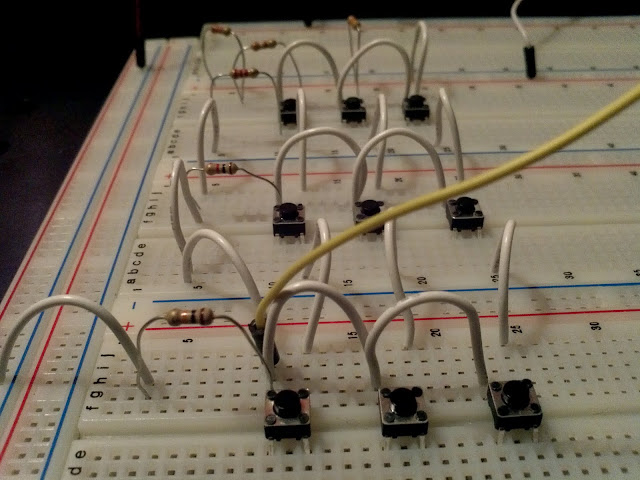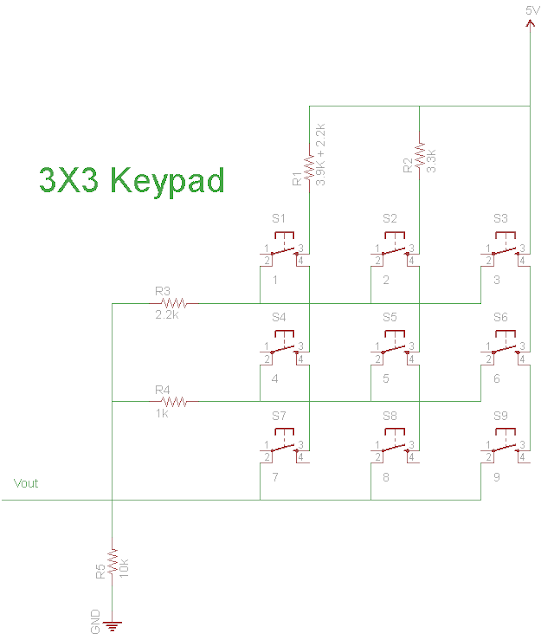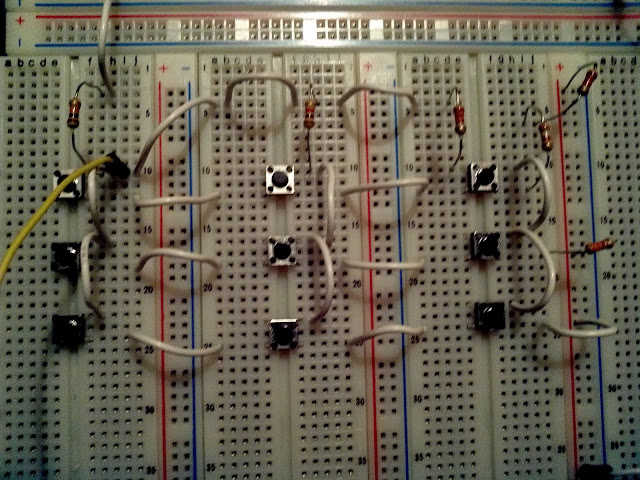# One Ouput Keypad Circuit Design

by on July 15, 2011

in CircuitsWhat can you do with a bunch of buttons and a few resistors? How about a keypad for your next microcontroller project.

The following is a 3×3 keypad that could easily be extended to more keys, when a button is pressed the resistors in that button’s column and row form a voltage divider with the 10k resistor below. The cool thing about this keypad is that it would only require one pin of a micronctroller.The output (Vout) is taken at the 10k resistor. The output can then be fed to a microcontroller A/D or any other circuit that can read different voltages, because each button produces a unique combination of resistors the output will be different for every button.

In my original design I had a 6k and 2k resistors, these were replaced with 3.9K and 2.2k and 2.2k respectively because I did not have 6k nor 2k in my resistor box. A picture of the circuit in real life is shown below.keypad circuit in breadboard. The picture was taken sideways, from left to right and top to bottom the keys are 7,4,1;8,5,2;9,6,3

Using a 10bit A/D converter the output of this keypad is the following.

Key Bit Value In Decimal Analog (V) Analog Expected(V)
1 554-556 2.71-2.77 2.7322
2 650-662 3.18-3.24 3.23
3 835-840 4.08-4.11 4.10
4 595-602 2.91-2.94 2.92
5 711-716 3.48-3.50 3.50
6 929-931 4.54-4.55 4.55
7 631-637 3.08-3.11 3.11
8 766-770 3.74-3.76 3.76
9 1023 5.0 5.0

Note that because the resistors actual value vary so does the output but they still fall within a range that is different for each button. The bit value to analog conversion was done using the formula

```[VoltageSource/(2^numberOfBitsOfA/D)]*BitValueOfKey=Analog
```

In our circuit the formula,assuming a 10 bit A/D converter, translates to

```[5V/(2^10)]*BitValueOfKey=Analog
```

The expected analog value was calculated using the voltage divider formula for each button where

```Vbutton=VSource*10k/(Rrow+Rcolumn+10k)
```

## Possible Microcontroller Implementation

If you want to implement this keypad in a microcontroller connect its output to an analog pin of the micro and use the following conditions in a function to differentiate the keys, note that I left more margin for possible errors from the readings above.

```  if(voltage>=550 && voltage<=570)
return 1;
else if(voltage>=645 && voltage<670)
return 2;
else if(voltage>=830 && voltage<=845)
return 3;
else if(voltage>=590 && voltage<=610)
return 4;
else if(voltage>=700 && voltage<=725)
return 5;
else if(voltage>=920 && voltage<=940)
return 6;
else if(voltage>=620 && voltage<=640)
return 7;
else if(voltage>=760 && voltage<=775)
return 8;
else if(voltage>=1000 && voltage<=1023)
return 9;
```

How can we improve this circuit? leave your comments or questions below.